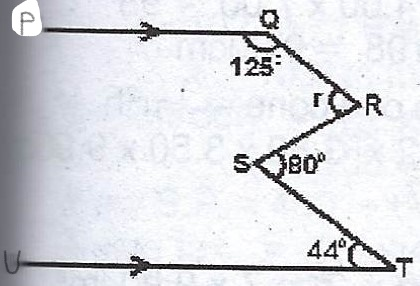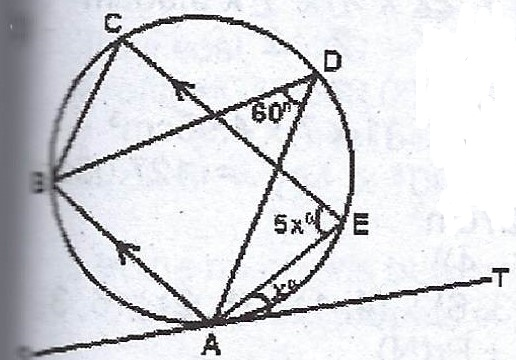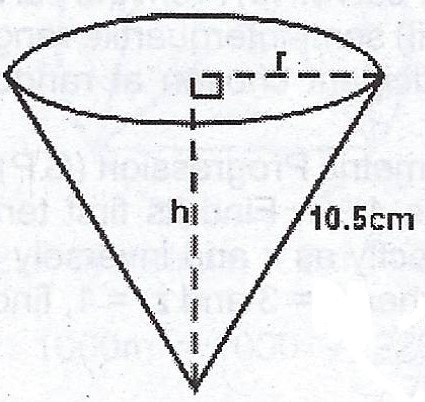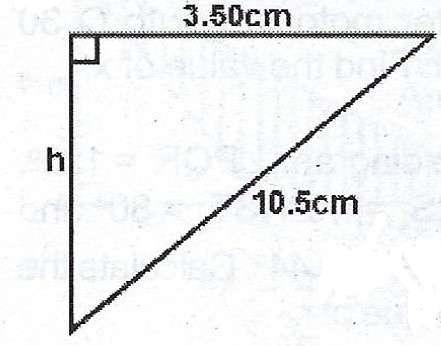### Mathematics Past Questions

3711

A = {2, 4, 6, 8}, B = {2, 3, 7, 9} and C = {x : 3 < x < 9} are subsets of the universal set U = {2, 3, 4, 5, 6, 7, 8, 9}. Find

(a) $$A \cap (B' \cap C')$$ ;

(b) $$(A \cup B) \cap (B \cup C)$$.

3712

(a) The angle of depression of a boat from the mid-point of a vertical cliff is 35°. If the boat is 120m from the foot of the cliff, calculate the height of the cliff.

(b) Towns P and Q are x km apart. Two motorists set out at the same time from P to Q at steady speeds of 60 km/h and 80 km/h. The faster motorist got to Q 30 minutes earlier than the other. Find the value of x.

3713

(a)In the diagram, < PQR = 125°, < QRS = r, < RST = 80° and < STU = 44°. Calculate the value of r.

(b)In the diagram TS is a tangent to the circle at A. AB // CE, < AEC = 5x°, < ADB = 60° and < TAE = x. Find the value of x.

3714

(a)Curved Surface Area = $$\pi rl$$

$$115.5 = \frac{22}{7} \times r \times 10.5$$

$$115.5 = 33r$$

$$r = \frac{115.5}{33} = 3.5 cm$$

(b)$$\therefore h^{2} + (3.50)^{2} = (10.5)^{2}$$

$$h^{2} = 10.5^{2} - 3.5^{2}$$

$$h^{2} = 98 \implies h = \sqrt{98}$$

$$h = 9.8994 cm \approxeq 9.90 cm$$

(c) Volume of a cone = $$\frac{1}{3} \pi r^{2} h$$

= $$\frac{1}{3} \times \frac{22}{7} \times \frac{7}{2} \times \frac{7}{2} \times 9.90$$

= $$\frac{23.1 \times 11}{2}$$

= $$127.05 cm^{3} \approxeq 127 cm^{3}$$

3715

Two fair die are thrown. M is the event described by "The sum of the scores is 10" and N is the event described by "The difference between the scores is 3".

(a) Write out the elements of M and N.

(b) Find the probability of M or N.

(c) Are M and N mutually exclusive? Give reasons.